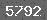# LED_MR16_3W热仿真MR16-3W Thermal simulation report
By Johnson
2010/03/31
https://www.resheji.com

Physical model decomposition diagram
Lamp holder
Power
LED
MCPCB
Heat sink
Lamppanel
Second optical lens
Screw
LED Lens
Die
Heatsink
Plastic frame
Pin

Note: The simulation model has been simplified to remove that do not affect the thermal performance of small features, such as screws, screw thread, small hole, round corner, chamfer, etc,in order to reduce the computing time.
Simulation model decomposition diagram
coordinate direction

Physical Features
Heat conduction in solids: On
Gravitational effects: On
Flow type: Laminar and turbulent
High Mach number flow: Off
Humidity: Off
Default roughness: 0 micrometer https://www.resheji.com
Gravitational Settings
Thermodynamic parameters
Static Pressure: 101325 Pa
Temperature: 35 °C
Velocity parameters
Velocity vector
Velocity in X direction: 0 m/s
Velocity in Y direction: 0 m/s
Velocity in Z direction: 0 m/s
Solid parameters
Initial solid temperature: 36°C
Turbulence parameters
Turbulence intensity and length
Intensity: 0.1 %
Length: 0.000493m
Ambient Conditions

Material Settings
Components
Material
Fluids
Air
Lamp holder
ABS
Power
Typical TQFP
Heat sink
Aluminum
MCPCB
Aluminum5052
LEDLens
Silicone
Die
Silicon Carbide
Pin
Aluminum
Plastic frame
PPA
Lamppanel
Aluminum
Second optical lens
PMMA
Heat sources
Single LED Die:1W
Total:3x1=3W

Results
Name  Temperature (℃)
Tj1   90.4
Tj2   90.4
Tj3  91.2
Tfin  77.2

Thermal resistance
Note:
Tj -----LED junction temperature
Tfin -----Heat sink fin temperature
Ta -----Ambient temperature
Rj-a -----Junction-to-ambient thermal resistance
Rj-a=(T j-Ta)/W=(90.7-35)/3=18.57 (℃/W)
Temperature field  Mesh

Plot Velocity field  Section location  Temperature field

Plot Mesh Velocity field Section location

Conclusion
When the ambient temperature is 35 ℃,the temperature of LED junction is 90.7 ℃,and the design value of standard junction temperature is 100 ℃,so the designs of this lamps accord with the thermal design requirement. https://www.resheji.com

Accessories
Gases:
Air
Specific heat ratio (Cp/Cv): 1.399
Molecular mass: 0.02896 kg/mol
Dynamic viscosity
Dynamic viscosity[Pa*s]
Temperature[°C]
Specific heat (Cp)
Specific heat (Cp)[J/(kg*K)]
Temperature[°C]
Thermal conductivity
Thermal conductivity[W/(m*K)]
Temperature[°C]

Solids
Aluminum
Density: 2688.9 kg/m^3
Conductivity type: Isotropic
Melting temperature: 660.25 °C
Specific heat[J/(kg*K)]
Temperature[°C]
Specific heat

Thermal conductivity[W/(m*K)]
Temperature[°C]
Thermal conductivity
Resistivity[Ohm*m]
Temperature[°C]
Resistivity

Aluminum 5052
Density: 2680 kg/m^3
Specific heat: 921 J/(kg*K)
Conductivity type: Isotropic
Resistivity: 4.9e-08 Ohm*m
Melting temperature: 607.23 °C
Thermal conductivity[W/(m*K)]
Temperature[°C]
Thermal conductivity

Silicon Carbide
Density: 3000 kg/m^3
Specific heat: 710 J/(kg*K)
Conductivity type: Isotropic
Melting temperature: 2700 °C
Thermal conductivity
Thermal conductivity[W/(m*K)]
Temperature[°C]

Density: 1050 kg/m^3
Specific heat: 2050 J/(kg*K)
Conductivity type: Isotropic
Thermal conductivity: 0.189 W/(m*K)
Melting temperature: 135 °C

Typical TQFP
Density: 2000 kg/m^3
Specific heat: 800 J/(kg*K)
Conductivity type: Isotropic
Thermal conductivity: 0.2 W/(m*K)
Melting temperature: 1415.05 °C

PPA(Polyphthalamide)
Density: 1490 kg/m^3
Specific heat: 1968 J/(kg*K)
Conductivity type: Isotropic
Thermal conductivity: 0.49 W/(m*K)
Melting temperature: 311°C

Silicone
Density: 1210 kg/m^3
Specific heat: 1460 J/(kg*K)
Conductivity type: Isotropic
Thermal conductivity: 0.423W/(m*K)
Melting temperature: 316 °C

Density: 1170 kg/m^3
Specific heat: 1430 J/(kg*K)
Conductivity type: Isotropic
Thermal conductivity: 0.207W/(m*K)
Melting temperature: 146 °C

FloEFD资料下载:  LED_MR16_3W热仿真.pdf

 留言与评论（共有 0 条评论）

 验证码：